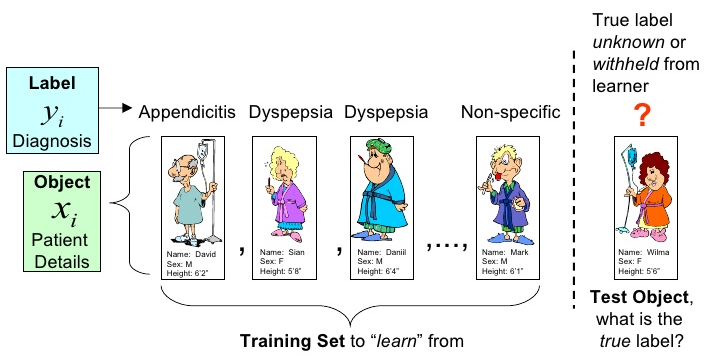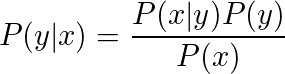# Data mining with Machine Learning for the social sciences

## Introduction, Challenges, the right & the wrong, Misunderstanding

 Priv.-Doz. Dr. Stefan Bosse University of Koblenz-Landau, Fac. Computer Science University of Bremen, Dept. Mathematics & Informatics 18.5.2018 sbosse@uni-bremen.de

## Introduction to Artificial Intelligence

### Artificial Intelligence

In social science big data volumes must be handled.
But big do not mean helpful or important!
Data is noisy and uncertain!?

• One major task in data science is the derivation of fundamental mapping functions:

F(Input Data): Input Data Output Data

F(Sensor Data): Sensor Data Knowledge

• Such a function F performs Feature Extraction

• But often there are no or only partial numerical/mathematical models that can implement F!

### Artificial Intelligence

• Usage of Artificial Intelligence and their methods can be helpful to derive such fundamental mapping functions - or at least an approximation: Hypothesis

• The input data is characterized commonly by a high dimensionality consisting of a vector of variables

[x1,x2,..,xn],

• whereby the output data (information) has a much lower dimensionality (data reduction!) consisting of the variable vector

[y1,y2,..,ym]

• This means:

F: RN RM with M N

• Data reduction includes the pre-selection of suitable (high information entropy) data variables Feature Selection

### Machine Learning - Technical Sciences

• Often there are no functional relations between two variables x and y.
In technical applications x can be a camera image with 1 Million pixels and y a figure from the set {0,1,2,..,9} that represent a hand written character. Generally:

f(x):x y.

• Machine Learning (ML) can be used to derive such relation from experimental/empirical training data!

• Among the derivation of such functional relations the prediction of what will happen next or in the future is an important task of Machine Learning

### Machine Learning - The Functional Approach

• Machine learning means the derivation of a hypothesis of a simple input-output function from training data provided by humans (statistical data!)!

### Machine Learning - Medicine

#### Diagnosis of Appendicitis from medicine and personal data

Input Data x

Patient Details [weight,age,sex,pain left, pain right, temperature, ..]

Output Data y

Diagnosis Label {Appendicitis, Dyspepsia, Unknown, .. }

Decision Learner

Returns one of the labels matching a new input vector x (the test object)### Machine Learning - Medicine

• Decision classifiers only return one (good or bad) matching label

• No information about matching probability

Probalistic Learner (Bayes Theorem)
Feature: Probability forecast estimating the conditional probability of best matching (or all) label(s) with a given observed object x